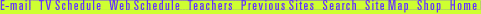## Demonstrate the Pythagorean Theorem

Think of each side of a right triangle as also being a side of a square that's attached to the triangle. The area of a square is any side multiplied by itself. (For example, a x a = a2.)

On the diagram below, show that a2 + b2 = c2, by moving the two small squares to cover the area of the big square.

How can I use the Pythagorean theorem to solve real problems?

Andrew Wiles | Math's Hidden Woman | Pythagorean Puzzle
Resources | Guide | Transcript | Proof Home